Question

# A 250V, 100 Hz sinusoidal supply is connected to a parallel network comprising three branches A,...

A 250V, 100 Hz sinusoidal supply is connected to a parallel network comprising three branches A, B, and C as follows: A, a coil of resistance 10 ohms; B, a series circuit of 5 ohms and inductive reactance of 9 ohms; C, a series circuit of 6 ohms, inductance of 50mH, and a capacitive reactance of 10 ohms. Determine

a) The apparent power of the combination

b) The rating and value of the capacitor to be connected in parallel to raise the power factor to 89%

Guys plss rush :(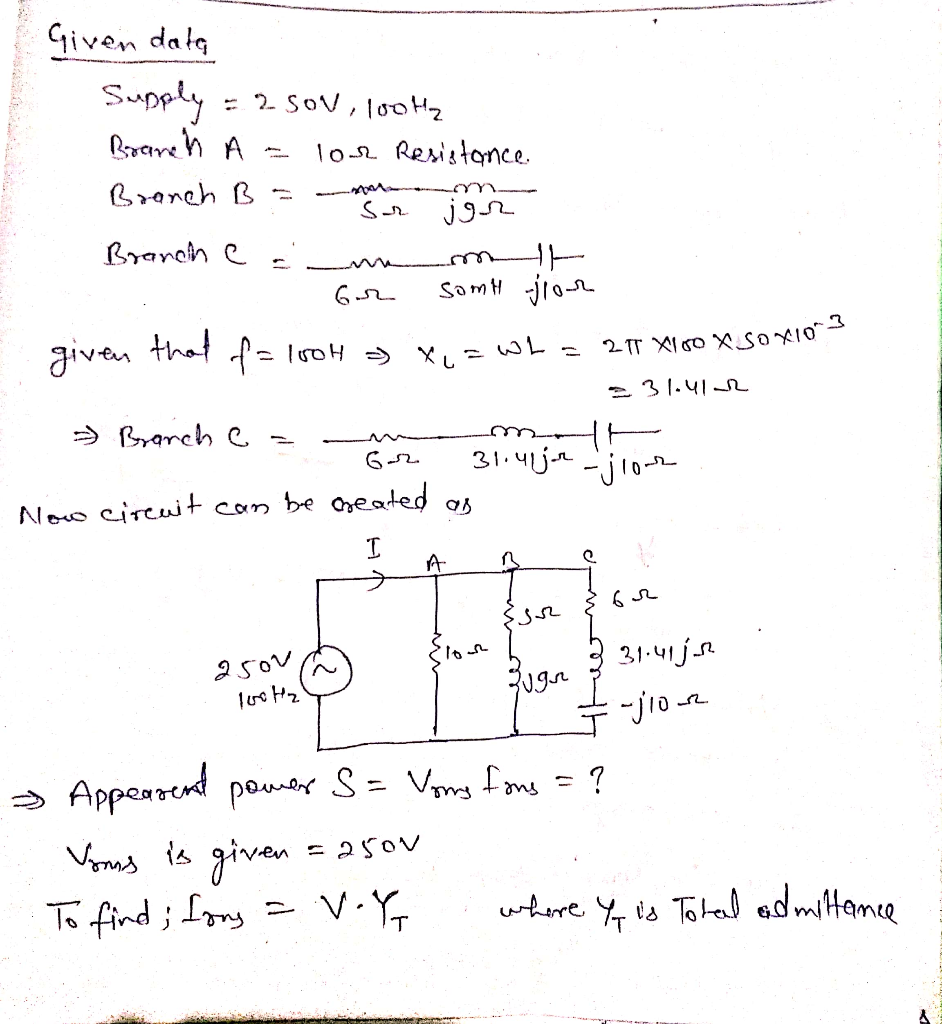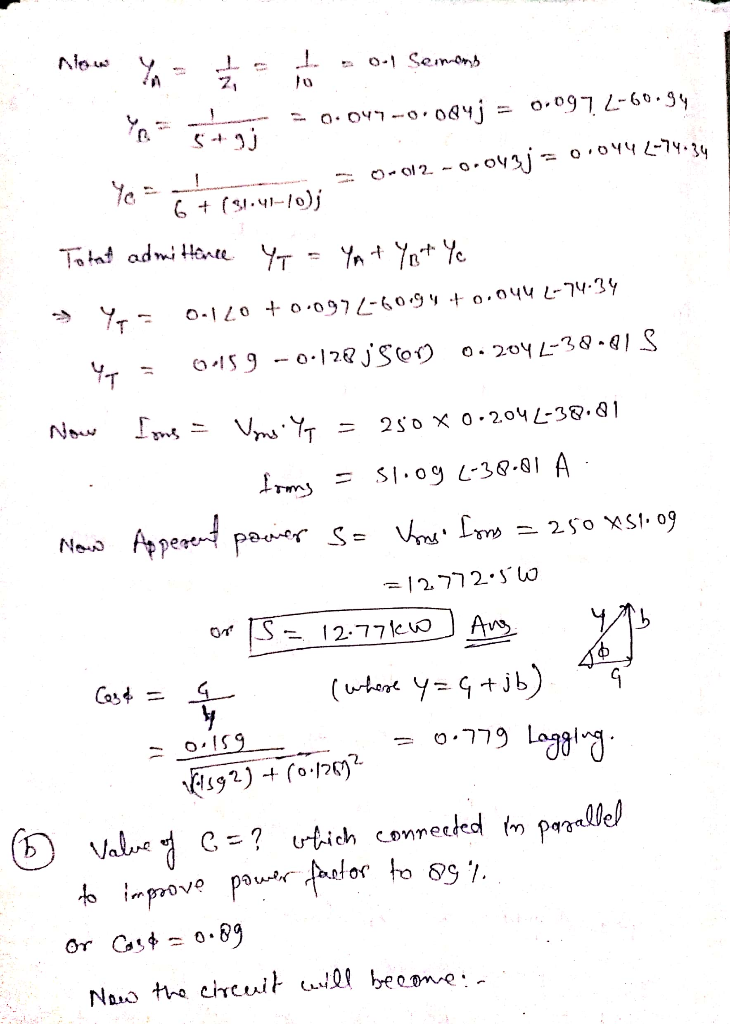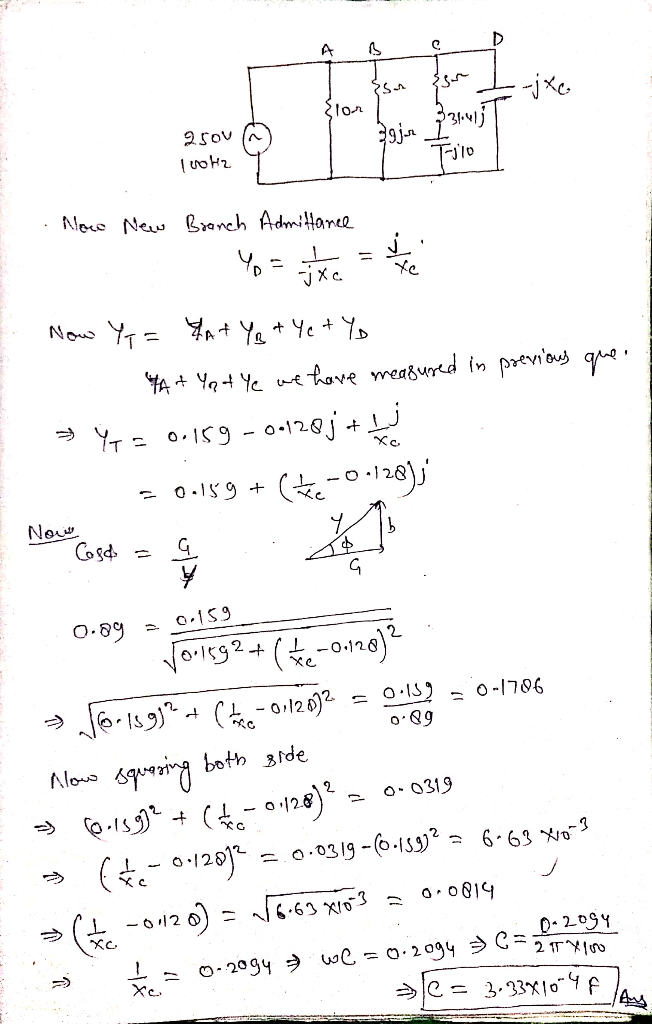#### Earn Coins

Coins can be redeemed for fabulous gifts.

Similar Homework Help Questions
• ### Two circuit Aand B are connected to a 115V, 50Hz supply. The total current taken by...

Two circuit Aand B are connected to a 115V, 50Hz supply. The total current taken by combination is 10 Amps at unity power factor. Circuit A consists of a 10 ohms resistor and a 200 uf capacitor connected in series, circuit B consists of a resistor and an inductive in series. Determine the current, the impedance, resistance and reactance

• ### Alternating Current Circuits Containing Inductance Cirele the letter beside the correct answer Three inductors connected in...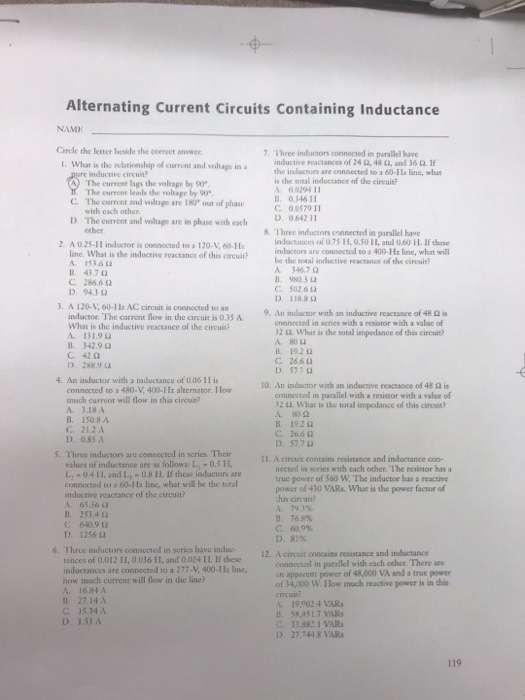Alternating Current Circuits Containing Inductance Cirele the letter beside the correct answer Three inductors connected in parallel have inductive reactances of 24 Ω, 48 Ω, and 36 Ω. If the inductors are connected to a 60-He line, wha is the sotal inductance of the eircui? A. 00294 11 , 0.34611 C 0 0579 11 D. 0642 11 I. What is the relationship of current and voltage in a inductive circuit The currene lags the voltage by 90 The current leads...

• ### 2. An AC power source with V 220 V and f60.0 Hz is connected in a...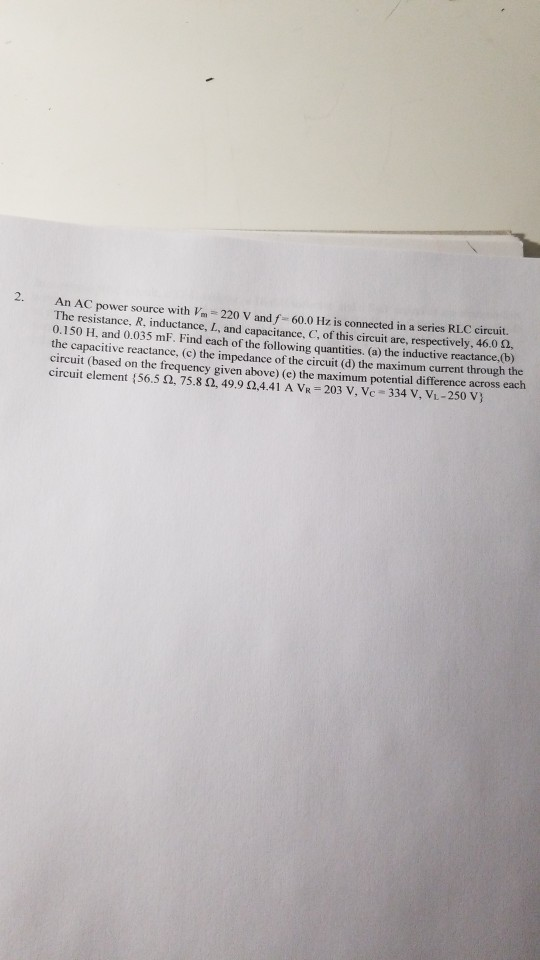2. An AC power source with V 220 V and f60.0 Hz is connected in a series RLC circuit. The resistance, R. inductance, L, and capacitance. C. of this circuit are, respectively. 46.0 12, 0.150 H. and 0.035 mF. Find each of the following quantities, (a) the inductive reactance, the capacitive reactance, (c) the impedance of the circuit (d) the maximum current through the circuit (based on the frequency given above) (e) the maximum potential difference across each circuit element...

• ### I just need answers to f,g,and h connected motor is connected to Vi-L 120 3T/6 V-rms, 50 Hz three e resistance and inductance of each phase is 8-Ω and 0.04 H, respectively. The PS. A three-phase p...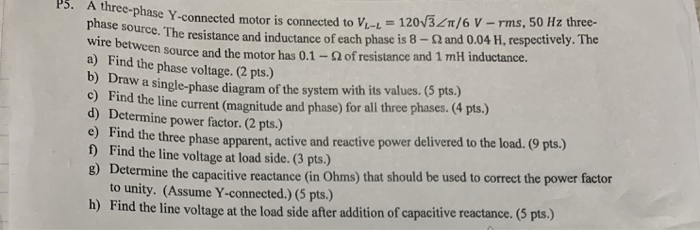I just need answers to f,g,and h connected motor is connected to Vi-L 120 3T/6 V-rms, 50 Hz three e resistance and inductance of each phase is 8-Ω and 0.04 H, respectively. The PS. A three-phase phase source. The wire between a source and the motor has 0.1-Ω of resistance and 1 mH inductance. nd the phase voltage. (2 pts.) a single-phase diagram of the system with its values. (5 pts.) c) Find the line current (magnitude and phase) for...

• ### Two electrical loads are connected in parallel to a 380 V, 50 Hz, three phase supply....

Two electrical loads are connected in parallel to a 380 V, 50 Hz, three phase supply. The first load consists of three identical impedance each 7 Ω resistance and 5Ω inductive resistance, connected in star. The second load consists of three identical impedances, each 12 Ω resistance and 8 Ω capacitive reactance connected in delta. Sketch the arrangement described above Calculate Zph1 and Zph2[Zph1= 8.6Ω 35.54°; Zph2= 14.42 Ω -33.69°] Determine Iph1 and Iph2[Iph1= 25.51A -35.54°; Iph2= 26.35A +33.69°] IL1...

• ### A three-phase Yy0 connected transformer has a nominal rating of 250 kVA and a voltage ratio...

A three-phase Yy0 connected transformer has a nominal rating of 250 kVA and a voltage ratio 15:0.4 kV. After conducting the short-circuit test, where the secondary winding is short-circuited, it is found that the primary series resistance is 0.08 pu and the primary series impedance magnitude is 0.1pu. The effect of the parallel branch in the transformer’s equivalent circuit may be neglected. Explain what information ‘Yy0’ gives about the transformer. Draw the per-phase equivalent circuit of the transformer during the...

• ### Course and Section cto EXPERIMENT ac series-Parallel Sinusoidal Circuits OBJECTIVES 1. Measure th...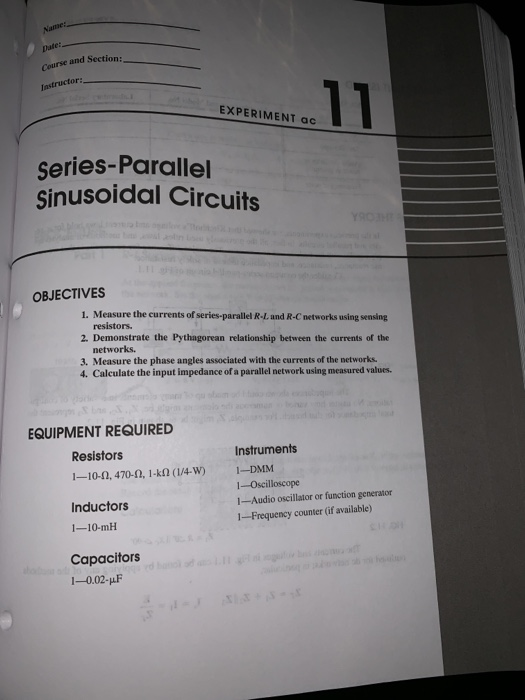Course and Section cto EXPERIMENT ac series-Parallel Sinusoidal Circuits OBJECTIVES 1. Measure the currents of series-parallel R-L and R-C networks using sensing resistors 2. Demonstrate the Pythagorean relationship between the currents of the networks. 3. Measure the phase angles associated with the currents of the networks. 4. Calculate the input impedance of a parallel network using measured values EQUIPMENT REQUIRED Instruments Resistors 1-10-Q, 470-Ω, l-kM (14.W) Inductors 1-10-mH Capacitors 1-0.02-pF I-DMM 1--Oscilloscope 1-Audio oscillator or function generator 1--Frequency counter (if...

• ### 1) An inductor of 45 mH that has a 2.6 mA current when connected to a...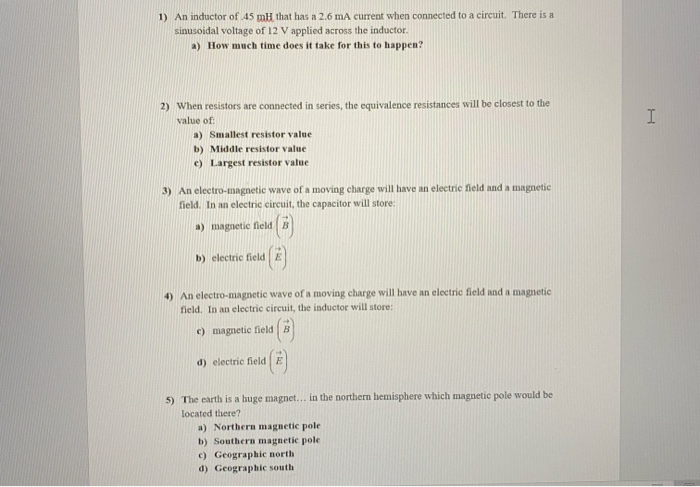1) An inductor of 45 mH that has a 2.6 mA current when connected to a circuit. There is a sinusoidal voltage of 12 V applied across the inductor. a) How much time does it take for this to happen? I 2) When resistors are connected in series, the equivalence resistances will be closest to the value of a) Smallest resistor value b) Middle resistor value c) Largest resistor value 3) An electro-magnetic wave of a moving charge will have...